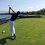# Another factoring trick

The other day, while helping my friend on homework, I was stuck on a problem. And by stuck I mean I didn't want to guess rational solutions and then synthetic to find if they worked because that's too main stream and I had already done that to change it from a 4th degree to a third.

So I just happened to come across a little trick which I'll detail in this note on how to factor Polynomials such as $2x^4-19x^3+57x^2-64x+20$.

Let me begin by saying: This trick isn't the best for two reasons: It doesn't work all that often, and it may be a waste of time. +it's already kinda similar to RR and remainder theorem.

But on the other hand, when it works, it can be very helpful.

We begin by synthetic division on the polynomial $2x^4-19x^3+57x^2-64x+20$ Divide the entire polynomial by $x-2$ and we get $2x^3-15x^2+27x-10=0$

Move the 10 over to the other side

$x(2x^2-15x+27)=10$

$x(2x+3)(x-9)=10$

From here, we prime factorize 10 to $1\cdot 2\cdot5$.

Assuming x is rational, it's quite clear that x=1 or 2 or 5 since we have an x in the front of our equation. Thus we can plug in x= 1,2,5 to see which work. After doing so, we find that 2 and 5 both work.

Now, you might be asking "well how is this quicker". And the thing is that $\textbf{you don't have to multiply and add everything out}$ all you have to do is check that each binomial is a factor of 10.

This can become especially helpful when the constant term $a_0$ becomes quite large and you are stuck pluging in large numbers which when raised to the 5th power such as $6^5$ can become quite strenuous to do by hand.

Here's another example

## Find all roots of $x^4+5x^2-29406$ given that $29406=13^2\cdot 2\cdot 87$

Move the factors to the right.

$x^2(x^2+5)=13^2\cdot 2 \cdot 87$

A little intuition\manipulation yields that $2\cdot87=13^2+5$

Looking at our expression now gives is that $x^2(x^2+5)=13^2(13^2+5)$

Now it's quite obvious that $\pm13$ are roots just by looking at the equation.

Thus after dividing our equation by $x^2-169$ we get

$(x^2-169)(x^2+2\cdot87)=0$

$(x-13)(x+13)(x-i\sqrt{174})(x+i\sqrt{174})$

This our answer is $\boxed{x={\pm13,\pm i\sqrt{174}}}$Note by Trevor Arashiro
6 years, 7 months ago

This discussion board is a place to discuss our Daily Challenges and the math and science related to those challenges. Explanations are more than just a solution — they should explain the steps and thinking strategies that you used to obtain the solution. Comments should further the discussion of math and science.

When posting on Brilliant:

• Use the emojis to react to an explanation, whether you're congratulating a job well done , or just really confused .
• Ask specific questions about the challenge or the steps in somebody's explanation. Well-posed questions can add a lot to the discussion, but posting "I don't understand!" doesn't help anyone.
• Try to contribute something new to the discussion, whether it is an extension, generalization or other idea related to the challenge.

MarkdownAppears as
*italics* or _italics_ italics
**bold** or __bold__ bold
- bulleted- list
• bulleted
• list
1. numbered2. list
1. numbered
2. list
Note: you must add a full line of space before and after lists for them to show up correctly
paragraph 1paragraph 2

paragraph 1

paragraph 2

[example link](https://brilliant.org)example link
> This is a quote
This is a quote
    # I indented these lines
# 4 spaces, and now they show
# up as a code block.

print "hello world"
# I indented these lines
# 4 spaces, and now they show
# up as a code block.

print "hello world"
MathAppears as
Remember to wrap math in $$ ... $$ or $ ... $ to ensure proper formatting.
2 \times 3 $2 \times 3$
2^{34} $2^{34}$
a_{i-1} $a_{i-1}$
\frac{2}{3} $\frac{2}{3}$
\sqrt{2} $\sqrt{2}$
\sum_{i=1}^3 $\sum_{i=1}^3$
\sin \theta $\sin \theta$
\boxed{123} $\boxed{123}$

Sort by:

Solve It

$\large{1000x^3-1254x^2-496x+191}$

Solution

Let's make the substitution $x=\dfrac{y+1254}{3000}$ to delete the quadratic term: $1000\left(\dfrac{y+1254}{3000}\right)^3-1254\left(\dfrac{y+1254}{3000}\right)^2-496\left(\dfrac{y+1254}{3000}\right)+191=0$ Expand, clear denominators and simplify: $y^3-9181548y-4384726128=0$ Now, try to match that equation with the identity $(u+v)^3-3uv(u+v)-(u^3+v^3)=0$, an equation system will be formed: \quad \begin{aligned} y=u+v &\quad ...(1) \\ 3uv=9181548 &\quad ...(2) \\ u^3+v^3=4384726128 &\quad ...(3) \end{aligned} Divide $(2)$ by $3$ and cube both sides: $u^3v^3=28667113297167468096$ Now, make an equation in $z$ with roots $u^3$ and $v^3$: $(z-u^3)(z-v^3)=0 \Longrightarrow z^2-(u^3+v^3)z+u^3v^3=0$ $z^2-4384726128z+28667113297167468096=0$ Using the quadratic formula we get two values of $z$: $z=2192363064 \pm 6000i\sqrt{662796041466}$ Hence: $u=\sqrt{2192363064+6000i\sqrt{662796041466}}$ $v=\sqrt{2192363064-6000i\sqrt{662796041466}}$ Using complex numbers let's take the principal cube roots for $u$ and $v$: $u=\sqrt{|u|} cis \left(\dfrac{\arg u}{3}\right) \approx 1622.698958+653.730901i$ $v=\sqrt{|v|} cis \left(\dfrac{\arg v}{3}\right) \approx 1622.698958-653.730901i$ From the equation system, there are three possible values for $(u,v)$. They are: $(u,v)$, $(uw,vw^2)$ and $(uw^2,vw)$, where $w$ is any primitive 3rd root of unity. Can you check the other values that don't work? So, we have three solutions for $y$: $y_1=u+v \approx 3245.397916$ $y_2=uw+vw^2 \approx -2754.994093$ $y_3=uw^2+vw \approx -490.4038231$ Hence: $x_1=\dfrac{y_1+1254}{3000} \approx 1.4997$ $x_2=\dfrac{y_2+1254}{3000} \approx -0.5003$ $x_3=\dfrac{y_3+1254}{3000} \approx 0.2545$

$\Large{Credit~goes~to}$ @Alan Enrique Ontiveros Salazar

- 6 years, 7 months ago

I knew about this! Its a method to solve cubic! Right? I read it from "Hall and Knight". You might like to refer it.

- 6 years, 7 months ago

Thanks Trevor!

- 6 years, 7 months ago